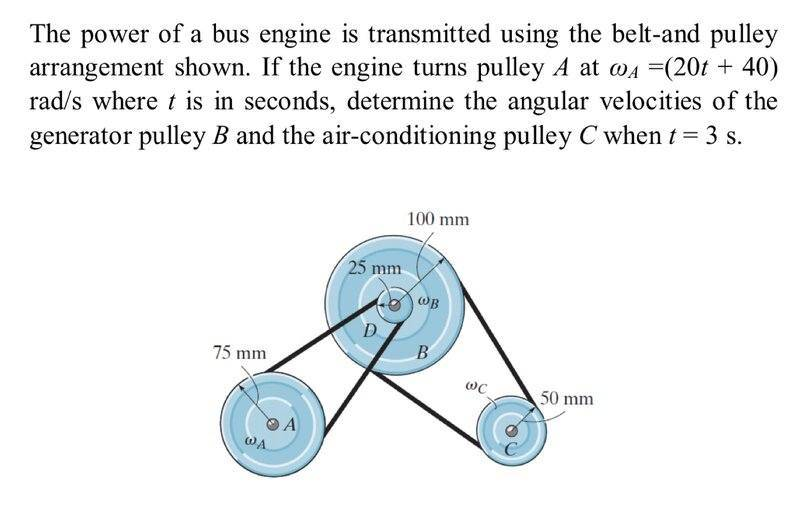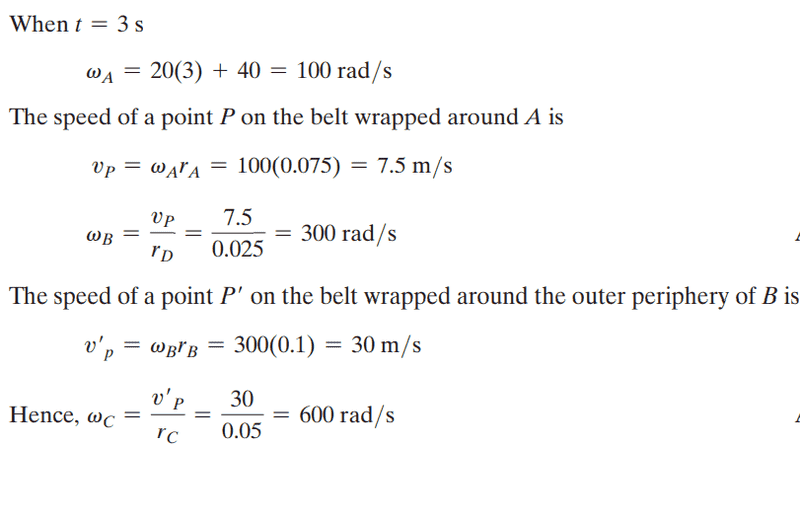# Angular Velocity: Pulley and belt system

thatstheguy9
Homework Statement:
Determine the angular velocity of Pulley B and C
Relevant Equations:
## V = \omega r ##So far I have:

The velocity of the belt will be the same for pully A and D, so we can calculate the angular velocity of pulley D:

## V_A = V_B ##
## \omega_A r_A = \omega_D r_D ##
## ((20*3)+40)(0.075) = \omega_D (0.025) ##
## \omega_D = 300 Rad/s ##

My next step was to determine the angular velocity of pulley B. My thought was because pully B and pulley D are joined on the same shaft they must have the same velocity.
I determined ## \omega_B = 75 Rad/s ##.
However the solution found ## \omega_B = 300 Rad/s ##.
I don't understand how the larger pulley can have a higher velocity than the smaller pulley. Or why the velocity of pulley A is the same as pulley B. Can someone explain this?Last edited:

Homework Helper
Gold Member
2022 Award
Homework Statement:: Determine the angular velocity of Pulley B and C
Relevant Equations:: ## V = \omega r ##

View attachment 290561

So far I have:

The velocity of the belt will be the same for pully A and D, so we can calculate the angular velocity of pulley D:

## V_A = V_B ##
## \omega_A r_A = \omega_D r_D ##
## ((20*3)+40)(0.075) = \omega_D (0.025) ##
## \omega_D = 300 Rad/s ##

My next step was to determine the angular velocity of pulley B. My thought was because they are joined on the same shaft they must have the same velocity.
I determined ## \omega_D = 75 Rad/s ##.
However the solution found ## \omega_D = 300 Rad/s ##.
I don't understand how the larger pulley can have a higher velocity than the smaller pulley. Or why the velocity of pulley A is the same as pulley B. Can someone explain this?
View attachment 290562
You seem to have got yourself confused.
You found ## \omega_D = 300 Rad/s ##, but then changed it to ## \omega_D = 75 Rad/s ##

thatstheguy9
You seem to have got yourself confused.
You found ## \omega_D = 300 Rad/s ##, but then changed it to ## \omega_D = 75 Rad/s ##
Apologies, I've corrected the original post.

Homework Helper
Gold Member
2022 Award
because they are joined on the same shaft
Which two are?

thatstheguy9
Which two are?
Pulley B and D

Homework Helper
Gold Member
2022 Award
Pulley B and D
Ok, so if D's angular velocity is 300r/s what is B's?

thatstheguy9
Ok, so if D's angular velocity is 300r/s what is B's?

## V_D = V_B ##
## \omega_D r_D = \omega_B r_B ##
## 300*0.025 = \omega_B * 0.100 ##

Homework Helper
Gold Member
2022 Award
## V_D = V_B##
Ok, I see. You posted
My next step was to determine the angular velocity of pulley B. My thought was because pully B and pulley D are joined on the same shaft they must have the same velocity.
So I thought you meant they must have the same angular velocity. Which is true.

thatstheguy9
Ok, I see. You posted

So I thought you meant they must have the same angular velocity. Which is true.
How can they have the same angular velocity when they have different radi?
Which brings me to my next point of confusion:
Why they have used the radius of pulley D to calculate the angular velocity of pulley B.

Homework Helper
Gold Member
2022 Award
How can they have the same angular velocity when they have different radi?
They're attached rigidly to the same axle. If the axle completes one turn, how many turns, or what fraction of a turn, has each pulley turned?

Homework Helper
Gold Member
How can they have the same angular velocity when they have different radi?
...
Perhaps you are confusing tangential and angular velocitires.

thatstheguy9
•Lnewqban